# Walkthrough: Matrix Multiplication

This step-by-step walkthrough demonstrates how to use C++ AMP to accelerate the execution of matrix multiplication. Two algorithms are presented, one without tiling and one with tiling.

## Prerequisites

Before you start:

• Make sure that Windows 7, Windows 8, Windows Server 2008 R2, or Windows Server 2012 is installed on your computer.

### To create the project

1. On the menu bar in Visual Studio, choose File, New, Project.

2. Under Installed in the templates pane, select Visual C++.

3. Select Empty Project, enter MatrixMultiply in the Name box, and then choose the OK button.

4. Choose the Next button.

5. In Solution Explorer, open the shortcut menu for Source Files, and then choose Add, New Item.

6. In the Add New Item dialog box, select C++ File (.cpp), enter MatrixMultiply.cpp in the Name box, and then choose the Add button.

## Multiplication without tiling

In this section, consider the multiplication of two matrices, A and B, which are defined as follows:A is a 3-by-2 matrix and B is a 2-by-3 matrix. The product of multiplying A by B is the following 3-by-3 matrix. The product is calculated by multiplying the rows of A by the columns of B element by element.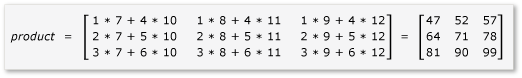### To multiply without using C++ AMP

1. Open MatrixMultiply.cpp and use the following code to replace the existing code.

``````#include <iostream>

void MultiplyWithOutAMP() {

int aMatrix = {{1, 4}, {2, 5}, {3, 6}};
int bMatrix = {{7, 8, 9}, {10, 11, 12}};
int product = {{0, 0, 0}, {0, 0, 0}, {0, 0, 0}};

for (int row = 0; row < 3; row++) {
for (int col = 0; col < 3; col++) {
// Multiply the row of A by the column of B to get the row, column of product.
for (int inner = 0; inner < 2; inner++) {
product[row][col] += aMatrix[row][inner] * bMatrix[inner][col];
}
std::cout << product[row][col] << "  ";
}
std::cout << "\n";
}
}

void main() {
MultiplyWithOutAMP();
getchar();
}
``````

The algorithm is a straightforward implementation of the definition of matrix multiplication. It does not use any parallel or threaded algorithms to reduce the computation time.

2. On the menu bar, choose File, Save All.

3. Choose the F5 keyboard shortcut to start debugging and verify that the output is correct.

4. Choose Enter to exit the application.

### To multiply by using C++ AMP

1. In MatrixMultiply.cpp, add the following code before the main method.

``````void MultiplyWithAMP() {
int aMatrix[] = { 1, 4, 2, 5, 3, 6 };
int bMatrix[] = { 7, 8, 9, 10, 11, 12 };
int productMatrix[] = { 0, 0, 0, 0, 0, 0, 0, 0, 0 };

array_view<int, 2> a(3, 2, aMatrix);
array_view<int, 2> b(2, 3, bMatrix);
array_view<int, 2> product(3, 3, productMatrix);

parallel_for_each(
product.extent,
[=](index<2> idx) restrict(amp) {
int row = idx;
int col = idx;
for (int inner = 0; inner < 2; inner++) {
product[idx] += a(row, inner) * b(inner, col);
}
}
);

product.synchronize();

for (int row = 0; row < 3; row++) {
for (int col = 0; col < 3; col++) {
//std::cout << productMatrix[row*3 + col] << "  ";
std::cout << product(row, col) << "  ";
}
std::cout << "\n";
}
}
``````

The AMP code resembles the non-AMP code. The call to parallel_for_each starts one thread for each element in product.extent, and replaces the for loops for row and column. The value of the cell at the row and column is available in idx. You can access the elements of an array_view object by using either the [] operator and an index variable, or the () operator and the row and column variables. The example demonstrates both methods. The array_view::synchronize method copies the values of the product variable back to the productMatrix variable.

2. Add the following include and using statements at the top of MatrixMultiply.cpp.

``````#include <amp.h>
using namespace concurrency;
``````
3. Modify the main method to call the MultiplyWithAMP method.

``````void main() {
MultiplyWithOutAMP();
MultiplyWithAMP();
getchar();
}
``````
4. Choose the Ctrl+F5 keyboard shortcut to start debugging and verify that the output is correct.

5. Choose the spacebar to exit the application.

## Multiplication with tiling

Tiling is a technique in which you partition data into equal-sized subsets, which are known as tiles. Three things change when you use tiling.

• You can create tile_static variables. Access to data in tile_static space can be many times faster than access to data in the global space. An instance of a tile_static variable is created for each tile, and all threads in the tile have access to the variable. The primary benefit of tiling is the performance gain due to tile_static access.

• You can call the tile_barrier::wait method to stop all of the threads in one tile at a specified line of code. You cannot guarantee the order that the threads will run in, only that all of the threads in one tile will stop at the call to tile_barrier::wait before they continue execution.

• You have access to the index of the thread relative to the entire array_view object and the index relative to the tile. By using the local index, you can make your code easier to read and debug.

To take advantage of tiling in matrix multiplication, the algorithm must partition the matrix into tiles and then copy the tile data into tile_static variables for faster access. In this example, the matrix is partitioned into submatrices of equal size. The product is found by multiplying the submatrices. The two matrices and their product in this example are: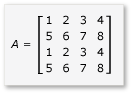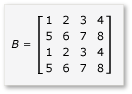The matrices are partitioned into four 2x2 matrices, which are defined as follows: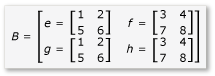The product of A and B can now be written and calculated as follows: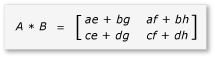Because matrices a through h are 2x2 matrices, all of the products and sums of them are also 2x2 matrices. It also follows that A*B is a 4x4 matrix, as expected. To quickly check the algorithm, calculate the value of the element in the first row, first column in the product. In the example, that would be the value of the element in the first row and first column of ae + bg. You only have to calculate the first column, first row of ae and bg for each term. That value for ae is 1*1 + 2*5 = 11. The value for bg is 3*1 + 4*5 = 23. The final value is 11 + 23 = 34, which is correct.

To implement this algorithm, the code:

• Uses a tiled_extent object instead of an extent object in the parallel_for_each call.

• Uses a tiled_index object instead of an index object in the parallel_for_each call.

• Creates tile_static variables to hold the submatrices.

• Uses the tile_barrier::wait method to stop the threads for the calculation of the products of the submatrices.

### To multiply by using AMP and tiling

1. In MatrixMultiply.cpp, add the following code before the main method.

``````void MultiplyWithTiling()
{
// The tile size is 2.
static const int TS = 2;

// The raw data.
int aMatrix[] =       { 1, 2, 3, 4, 5, 6, 7, 8, 1, 2, 3, 4, 5, 6, 7, 8 };
int bMatrix[] =       { 1, 2, 3, 4, 5, 6, 7, 8, 1, 2, 3, 4, 5, 6, 7, 8 };
int productMatrix[] = { 0, 0, 0, 0, 0, 0, 0, 0, 0, 0, 0, 0, 0, 0, 0, 0 };

// Create the array_view objects.
array_view<int, 2> a(4, 4, aMatrix);
array_view<int, 2> b(4, 4, bMatrix);
array_view<int, 2> product(4, 4, productMatrix);

// Call parallel_for_each by using  2x2 tiles.
parallel_for_each(product.extent.tile< TS, TS >(),
[=] (tiled_index< TS, TS> t_idx) restrict(amp)
{
// Get the location of the thread relative to the tile (row, col) and the entire array_view (rowGlobal, colGlobal).
int row = t_idx.local;
int col = t_idx.local;
int rowGlobal = t_idx.global;
int colGlobal = t_idx.global;
int sum = 0;

// Given a 4x4 matrix and a 2x2 tile size, this loop executes twice for each thread.
// For the first tile and the first loop, it copies a into locA and e into locB.
// For the first tile and the second loop, it copies b into locA and g into locB.
for (int i = 0; i < 4; i += TS) {
tile_static int locA[TS][TS];
tile_static int locB[TS][TS];
locA[row][col] = a(rowGlobal, col + i);
locB[row][col] = b(row + i, colGlobal);
// The threads in the tile all wait here until locA and locB are filled.
t_idx.barrier.wait();

// Return the product for the thread. The sum is retained across
// both iterations of the loop, in effect adding the two products
// together, for example, a*e.
for (int k = 0; k < TS; k++) {
sum += locA[row][k] * locB[k][col];
}

// All threads must wait until the sums are calculated. If any threads
// moved ahead, the values in locA and locB would change.
t_idx.barrier.wait();
// Now go on to the next iteration of the loop.
}

// After both iterations of the loop, copy the sum to the product variable by using the global location.
product[t_idx.global] = sum;
});

// Copy the contents of product back to the productMatrix variable.
product.synchronize();

for (int row = 0; row < 4; row++) {
for (int col = 0; col < 4; col++) {
// The results are available from both the product and productMatrix variables.
//std::cout << productMatrix[row*3 + col] << "  ";
std::cout << product(row, col) << "  ";
}
std::cout << "\n";
}

}
``````

This example is significantly different than the example without tiling. The code uses these conceptual steps:

1. Copy the elements of tile[0,0] of a into locA. Copy the elements of tile[0,0] of b into locB. Notice that product is tiled, not a and b. Therefore, you use global indices to access a, b, and product. The call to tile_barrier::wait is essential. It stops all of the threads in the tile until both locA and locB are filled.

2. Multiply locA and locB and put the results in product.

3. Copy the elements of tile[0,1] of a into locA. Copy the elements of tile [1,0] of b into locB.

4. Multiply locA and locB and add them to the results that are already in product.

5. The multiplication of tile[0,0] is complete.

6. Repeat for the other four tiles. There is no indexing specifically for the tiles and the threads can execute in any order. As each thread executes, the tile_static variables are created for each tile appropriately and the call to tile_barrier::wait controls the program flow.

7. As you examine the algorithm closely, notice that each submatrix is loaded into a tile_static memory twice. That data transfer does take time. However, once the data is in tile_static memory, access to the data is much faster. Because calculating the products requires repeated access to the values in the submatrices, there is an overall performance gain. For each algorithm, experimentation is required to find the optimal algorithm and tile size.

In the non-AMP and non-tile examples, each element of A and B is accessed four times from the global memory to calculate the product. In the tile example, each element is accessed twice from the global memory and four times from the tile_static memory. That is not a significant performance gain. However, if the A and B were 1024x1024 matrices and the tile size were 16, there would be a significant performance gain. In that case, each element would be copied into tile_static memory only 16 times and accessed from tile_static memory 1024 times.

2. Modify the main method to call the MultiplyWithTiling method, as shown.

``````void main() {
MultiplyWithOutAMP();
MultiplyWithAMP();
MultiplyWithTiling();
getchar();
}
``````
3. Choose the Ctrl+F5 keyboard shortcut to start debugging and verify that the output is correct.

4. Choose the space bar to exit the application.